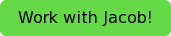#How to use this guide

This blog post is the first in a series on how to understand and approach kinematics problems. It is meant to supplement your class and textbook. I will focus on practical applications, how to solve problems, and common mistakes that students make. If you want to learn the basics of kinematics, I recommend a textbook, but if you want to gain a deeper understanding, avoid confusion and learn how to approach problems, take the red pill and join us!

# What is Kinematics?

Kinematics is simply the study of motion. This is literally what the word means: kinesis (motion) + tics (the study of. Think mathematics, politics, pizzatics). On a more practical level, the kinematics you learn in your intro physics class is the study of position, velocity, and momentum. On the most practical level, kinematics is the study of what happens when you toss a ball. Which means, if you can toss a ball, you can learn kinematics – FYI, as anyone who has ever seen me play sports knows, the reverse is not true.

# Position, Velocity, and Acceleration

So kinematics is the study of three things, but what exactly are those three things?

## Position:

Where an object is. It’s location. Physics problems will refer to this as x, y, z p, r, or d. Every teacher has their preferences, so learn yours, but for this blog we will use x and y. We are also often interested in the change in position, known as displacement, which is referred to as Δx, Δy, Δp, Δd or sometimes just x, y, or d to make things confusing. Position and displacement are usually measured in meters or m.

## Velocity:

How fast an object is moving and in what direction: aka how the distance is changing over time. Physics problems will almost always refer to velocity as v. Velocity is usually measured in meters per second, or m/s. When velocity is constant, it can be described by the equation v=Δx/Δt (the change in position divided by the change in time).

## Acceleration:

How the velocity of the object is changing. If a Ferrari goes from 0 to 60mph in 2.4 seconds then it is accelerating. If it the driver freaks out and crashes into a wall that stops the car, then it has also accelerated. Acceleration is always referred to as a, and is measured in meters per second per second, or m/s2.

When acceleration is constant, it can be is described by the equation a=Δv/Δt (the change in position divided by the change in time). You might notice that this equation is very similar to the equation for velocity. That is not a coincidence: it reflects the vast underlying physics that determines everything in the world.

# Avoid Common Mistakes: Position, Distance, and Displacement

Position, Displacement and Distance are easy to confuse and physics teachers often test to make sure you understand the difference. Position is measured from some origin point and defines the location of the object - in kinematics, we often define the origin point as the starting location of the object to make things easier. Distance measures the total distance an object has traveled. Displacement measures how far an object is from where it started.

To understand the difference, imagine a scenario where you start 3 meters to the right of the origin and walk 2 meters left, then 4 meters right. Your position at the beginning is 3 meters, and at the end it is 5 meters. Your distance is 6 meters because that’s how far you walked. Your displacement at the end, however, is 2 meters because you are only 2 meters away from where you started.# Avoid Common Mistakes: Velocity and Speed

In regular life we use the words velocity and speed interchangeably, but in physics we never do. Speed is how fast something moves, velocity is how fast it moves AND in what direction (we call this concept of magnitude+direction a vector). That means an object can change velocity without changing speed, for examples a car going around a circular track. It also means that speed can increase while velocity decreases. If velocity is negative and acceleration is negative then the velocity will be a more and more negative number, so velocity will decrease (go from -60 m/s to -120 m/s) but speed will increase (go from 60 m/s to 120 m/s).

# Avoid Common Mistakes: Acceleration and Velocity

Students new to physics are often puzzled by the idea that acceleration and velocity can go in different directions. When an object accelerates it should go faster, right? Not necessarily. For example, if you throw an object in the air, it starts with a positive, upward velocity but the acceleration is negative and downward. Eventually, the object stops going up and falls back to the ground, at which point the acceleration and the velocity are in the same direction. However, at any given moment the acceleration can be in any direction – acceleration determines the change in velocity NOT the velocity itself.

# Conclusion

So now we understand the basics of kinematics, but how do we use them? In my next blog, I will cover the standard equations of kinematics and how to use them to solve problems in 1-Dimension.

Stayed Tuned! Same bat time, same bat channel.

Are you interested in working with Jacob--either in New York or online--on your physics work?For more relevant reading, check out these other blog posts written by our physics tutors in New York and Boston:

Tags: physics